# Algebra - math word problems

1. Last pageTwo consecutive sheets dropped out of the book. The sum of the numbers on the sides of the dropped sheets is 154. What is the number of the last page of the dropped sheets?
2. Annual pensionCalculate the amount of money generating an annual pension of EUR 1000, payable at the end of the year and for a period of 10 years, shall be inserted into the bank to account with an annual interest rate of 2%
3. Twenty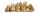Twenty rabbits are put in 4 cells so that there are different number of rabbits in each cell contains at least 3 rabbits. What is the largest possible number of rabbits in one cell
4. CarlaCarla is 5 years old and Jim is 13 years younger than Peter. One year ago, Peter’s age was twice the sum of Carla’s and Jim’s age. Find the present age of each one of them.
5. Two equationsSolve equations (use adding and subtracting of linear equations): -4x+11y=5 6x-11y=-5
6. Mixture 2How many liters of water must be added to 7 liters of a 20% solution to obtain a 10% solution?
7. Taxi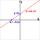A taxi ride costs \$3 plus \$2.50 per mile. Write and Graph an equation in two variables that represents the total cost of a taxi ride.
8. A large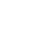A large gear will be used to turn a smaller gear. The large gear will make 75 revolutions per minute. The smaller gear must make 384 revolutions per minute. Find the smallest number of teeth each gear could have. [Hint: Use either GCF or LCM. ]
9. Area to perimeterCalculate circle circumference if its area is 254.34cm2
10. The volume 2The volume of a cube is 27 cubic meters. Find the height of the cube.
11. A perineumA perineum string is 10% shorter than its original string. The first string is 24, what is the 9th string or term?
12. Sand castle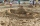Tim and Tom built a sand castle and embellished it with a flag. Half the pole with the flag plunged into the castle. The highest point of the pole was 80 cm above the ground, its lowest point 20 cm above the ground. How high was the sand castle?
13. Geometric progression 48,4√2,4,2√2
14. Rectangular trapezoidIn a rectangular trapezoid ABCD with right angles at vertices A and D with sides a = 12cm, b = 13cm, c = 7cm. Find the angles beta and gamma and height v.
15. Mba studiumAt MBA school, fourth-year students can choose from three optional subjects: a) mathematical methods, b) social interaction, c) management Each student studies one of these subjects. The mathematical methods studied 28 students, the social interaction 27.
16. EquatiomSolve equation with negatives: X/(-5) + 2 = -9
17. AlgebraX+y=5, find xy (find the product of x and y if x+y = 5)
18. EmployeesThe company employs 1 440 employees (men and women). For over-average results, the premiums were 18.75% of all men and 22.5% of all women. 20% of employees were rewarded with premiums. How many men and how many women are employed in the company?
19. Multiples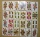What is the sum of the multiples of number 7 that are greater than 30 but less than 56?
20. LegsCancer has 5 pairs of legs. The insect has 6 legs. 60 animals have a total of 500 legs. How much more are cancers than insects?

Do you have an interesting mathematical word problem that you can't solve it? Enter it, and we can try to solve it.

To this e-mail address, we will reply solution; solved examples are also published here. Please enter the e-mail correctly and check whether you don't have a full mailbox.

Please do not submit problems from current active competitions such as Mathematical Olympiad, correspondence seminars etc...